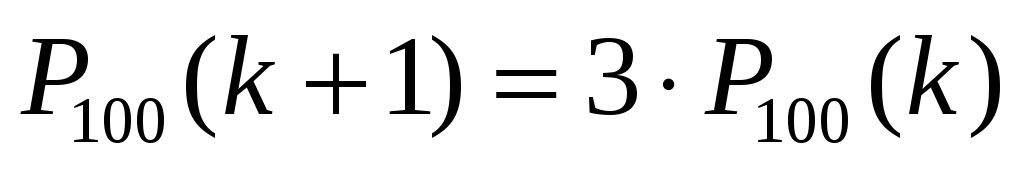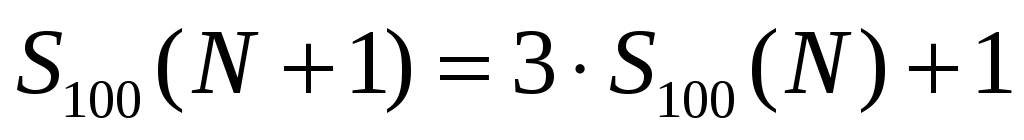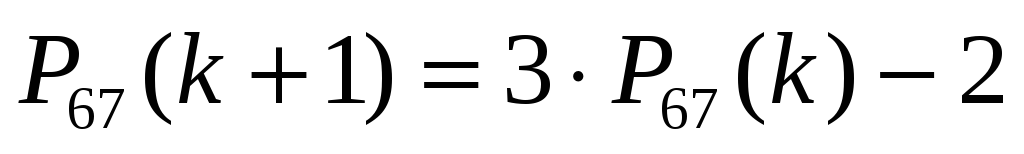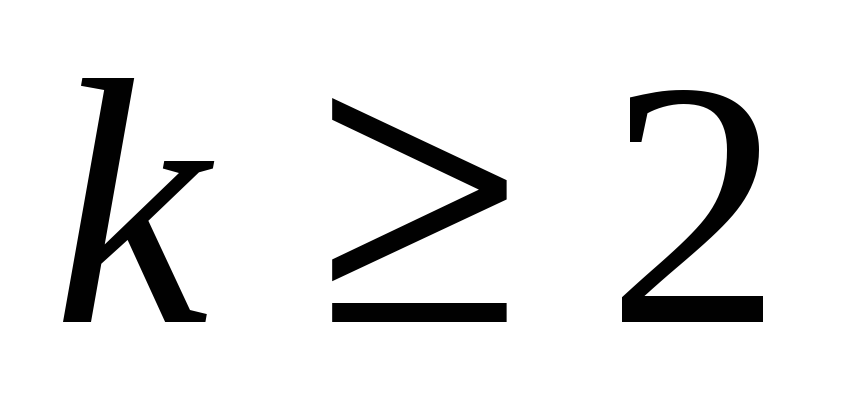# The Magnetic Tower of Hanoi

 Recursive Relations The four puzzle-solving algorithms discussed above follow remarkably simple (and thus elegant) recursive relations. This is true (recursive simplicity that is) for both the number of disk moves as well as for the total number of puzzle-solving moves. And in all relations, without exception, the leading term (on the right-hand side) is the product of the "current" value and the starring number - "3". Let's see. The "100" Algorithm:(22A)(22B) The "67" Algorithm:;(23A) Share with your friends: